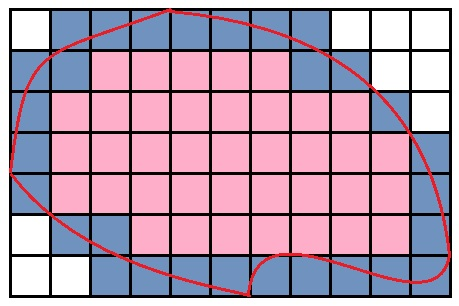# How to Approximate the Area of an Irregular Shape

There are many math formulas for computing the areas of simple geometric shapes, such as circles, triangles, and rectangles. But for most irregular shapes, there are no simple formulas to calculate area.

For irregular polygons (geometric shapes with straight edges) the most accurate way to compute the area is to partition the shape into triangles, then add up the areas of those smaller triangles.

For irregular shapes with curved edges, there are more crude ways to approximate the area, yet the results can still be quite accurate. The method described below requires only graph paper and two colored pencils.

First, copy the irregular shape onto a grid. Essentially, you will approximate the area of the irregular shape by estimating how many squares the shape covers on the grid paper. Make a note of the size or scaled size of each square. For example, a grid unit may be 1 square centimeter, inch, foot, or meter.

Next, color all of the squares that lie completely within the boundary of the shape and contain no part of the boundary line. Count them, and call the number of interior squares 'C'.

Use a different color to fill in the squares that contain the border of the shape. Only color in a square if the boundary line goes through the square. Count them, and call the number of perimeter squares 'P'.Now use the formula

Area = C + (0.5)P

to approximate the area of the shape. Example: suppose the number of interior squares is 38, and the number of boundary squares is 29. Using the approximation formula, we estimate the total area of the shape to be

38 + (0.5)(29) = 52.5

The finer the grid, i.e. the smaller the squares, the more accurate the estimate. Use this trick if you need to estimate the area of an irregularly shaped garden, pool, or piece of land.